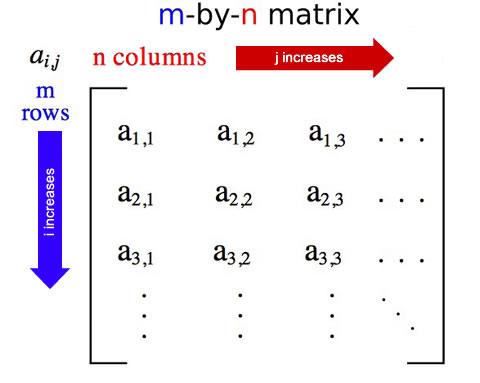## From C to C++

Since version 2.0 OpenCV introduced, in its new C++ API, the type cv::Mat, or simply Mat, to replace both C types CvMat and IplImage. Though both C structures are still supported, I'll highly recommend that you shift to C++. Why?

Here's why. Mat uniforms the concepts of matrix and image. In fact, they're the same thing in the end! Mat also adds some nice features such as the reference counter, that can be a great help. It takes off the burden of micromanaging memory yourself.

Other than this, you get some clean looking code too. Code that just makes sense.

## Introducing Mat

Before being able to use the C++ interface, you must "include" the OpenCV namespace. This is done by writing the following statement after the all #include statements:

```using namespace cv;
```

If you don't write this, you'll have to use cv:: to get access to things in this namespace, like cv::Mat.

The new Mat type supports matrix algebra in a “Matlab” style, for instance:

```Mat A=Mat(3,4,CV_32FC1);
Mat B=Mat(4,3,CV_32FC1);
...
//code for initialization of A and B
...
Mat C = 2*A*B;
```

Here C will be a Mat object representing a matrix of size 3x3, whose elements have been multiplied by the scalar (number) 2. This way of writing matrix algebra operations is much simpler and intuitive than using call to functions such as cvGEMM(...). And so it is inverting matrices using their method inv() or transposing them by using t().

```Mat C = C.inv(); //Now C is its own inverse matrix
Mat D = A.t(); //D is the transposed matrix of A
```

This brief introduction should be enough to understand why it is convenient to learn the new C++ interface.

## Internal structure

The Mat is the same as the old IplImage and CvMat structures. The "origin" is the top-left corner. Row and column numbers start from zero.The counter variable i varies from 0 to m-1. Similarly, the counter variable j varies from 0 to n-1.

## Matrix declaration

A matrix can be built in various formats. Defining this format is necessary (unlike other languages like Matlab or Python). The matrix can have one, two, three or four channels.

The most simple way to create a matrix is:

```Mat m = Mat(rows, cols, type);
```

The parameters are simple. rows and _cols _are the number of rows and columns in the matrix. _type _is the format of the matrix.

If you're creating an image, a more intuitive method of creating a Mat is:

```Mat m = Mat(Size(width,height), type);
```

And if you want to duplicate the size of another image, you can use:

```Mat n = Mat(m.size(), type);
```

type defines the number of bytes allocated for each element in the matrix. Check 2D matrices with CvMat in OpenCV for constants you can use here. Yes, constants remain the same for the C and C++ interface.

## Accessing elements

Accessing each pixel or element in a single channel Mat is trivial. You use the at method to access the value at a particular position (i, j).

```Mat a= Mat(4,3, CV_32FC1);
float elem_a= a.at<float>(i,j); //access element aij, with i from 0 to rows-1 and j from 0 to cols-1
```

Instead of specifying the position with i and j, you can use a Point object:

```Point p=Point(x,y);
float elem_a= a.at<float>(p); //Warning: y ranges from 0 to rows-1 and x from 0 to cols-1
```

If you're dealing with a multi-channel Mat, things are a little more complex. But still easier than the CV_MAT_ELEM or CV_IMAGE_ELEM macros. You must use the ptr method to obtain a pointer to a particular row. Then you use the [] to access a particular pixel in a particular channel:

```type elem = matrix.ptr<type>(i)[N~c~*j+c]
```

Here's what everything means:

• type: The datatype (float, int, uchar, etc)
• i: The row you're interested in
• Nc: The number of channels
• j: The column you're interested in
• c: The channel you're interested in (varies from 0 to 3)

This could be done for single channel image too, but offset will be always 0 and Nc always 1.

## Reshaping

Reshaping is playing around with the number of channels and the number of rows. Say we have a Nx1 matrix with Nc channels and we need to convert it into a NxNc matrix with 1 channel only. A simple reshape will help you accomplish this.

```Mat a= Mat(4,1, CV_32FC3); //a is 4x1, 3 channels
Mat b=a.reshape(1); //b is 4x3, 1 channel
```

Where would this be useful? Suppose you have a list of _Point _objects... something like this:

```vector<Point3f> v; //suppose it is already full
```

Suppose the list is already full with such points:

```[(x0, y0, z0)]
[(x1, y1, z1)]
[(x2, y2, z2)]
[(x3, y3, z3)]
[(..., ..., ...)]
```

You can "import" this list a matrix like this:

```Mat m1=Mat(v, true); //boolean value true is necessary in order to copy data from v to m1
```

If the boolean was not explicitly set to true, the matrix would only point to the list. It wouldn't be a true copy (i.e., duplication of data in memory).

So m1 has some rows (equal to v.size() ), exactly one column and three channels. You can reshape this matrix into a matrix with v.size() rows, 3 columns and one channel.Now, you can use use this reshaped matrix in algebraic equations (see above). This is often necessary when dealing with homogeneous coordinates.

## Some equivalents

Here's a list of C code and its equivalent in C++. It should help you port your code from C to C++

• CvSize -> Size
• CvVideoCapture -> VideoCapture
• IplImage, CvMat -> Mat
• cvQueryFrame -> >> (operator)
• cvShowImage -> imshow